# Assignments

In almost all applications of mathematics you need to be able to work with exponents. Whether the functions are root forms or fractions, you must always use a number of basic calculation rules. It is no less than a must to be able to apply these rules quickly. Learn them by heart and practice them with the following examples and assignments.

1. Simplify: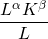Solution

2. Simplify: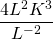Solution

3. Simplify: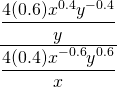Solution

4. Simplify: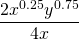Solution

5. Simplify: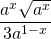Solution

6. Simplify: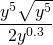Solution

7. Simplify: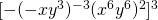Solution

8. Simplify: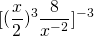Solution

9. Simplify: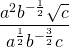Solution

10. Simplify:Solution

0